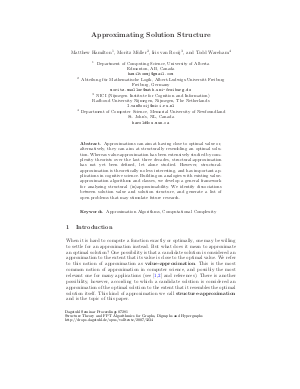Document# Approximating Solution Structure

### Authors Iris van Rooij, Matthew Hamilton, Moritz Müller, Todd Wareham## File

DagSemProc.07281.3.pdf
• Filesize: 261 kB
• 24 pages

## Cite As

Iris van Rooij, Matthew Hamilton, Moritz Müller, and Todd Wareham. Approximating Solution Structure. In Structure Theory and FPT Algorithmics for Graphs, Digraphs and Hypergraphs. Dagstuhl Seminar Proceedings, Volume 7281, pp. 1-24, Schloss Dagstuhl - Leibniz-Zentrum für Informatik (2007)
https://doi.org/10.4230/DagSemProc.07281.3

## Abstract

hen it is hard to compute an optimal solution \$y in optsol(x)\$ to an instance \$x\$ of a problem, one may be willing to settle for an efficient algorithm \$A\$ that computes an approximate solution \$A(x)\$. The most popular type of approximation algorithm in Computer Science (and indeed many other applications) computes solutions whose value is within some multiplicative factor of the optimal solution value, {em e.g.}, \$max(frac{val(A(x))}{optval(x)}, frac{optval(x)}{val(A(x))}) leq h(|x|)\$ for some function \$h()\$. However, an algorithm might also produce a solution whose structure is ``close'' to the structure of an optimal solution relative to a specified solution-distance function \$d\$, {em i.e.}, \$d(A(x), y) leq h(|x|)\$ for some \$y in optsol(x)\$. Such structure-approximation algorithms have applications within Cognitive Science and other areas. Though there is an extensive literature dating back over 30 years on value-approximation, there is to our knowledge no work on general techniques for assessing the structure-(in)approximability of a given problem. In this talk, we describe a framework for investigating the polynomial-time and fixed-parameter structure-(in)approximability of combinatorial optimization problems relative to metric solution-distance functions, {em e.g.}, Hamming distance. We motivate this framework by (1) describing a particular application within Cognitive Science and (2) showing that value-approximability does not necessarily imply structure-approximability (and vice versa). This framework includes definitions of several types of structure approximation algorithms analogous to those studied in value-approximation, as well as structure-approximation problem classes and a structure-approximability-preserving reducibility. We describe a set of techniques for proving the degree of structure-(in)approximability of a given problem, and summarize all known results derived using these techniques. We also list 11 open questions summarizing particularly promising directions for future research within this framework. vspace*{0.15in} oindent (co-presented with Todd Wareham) vspace*{0.15in} jointwork{Hamilton, Matthew; M"{u}ller, Moritz; van Rooij, Iris; Wareham, Todd}
##### Keywords
• Approximation Algorithms
• Solution Structure

## Metrics

• Access Statistics
• Total Accesses (updated on a weekly basis)
0